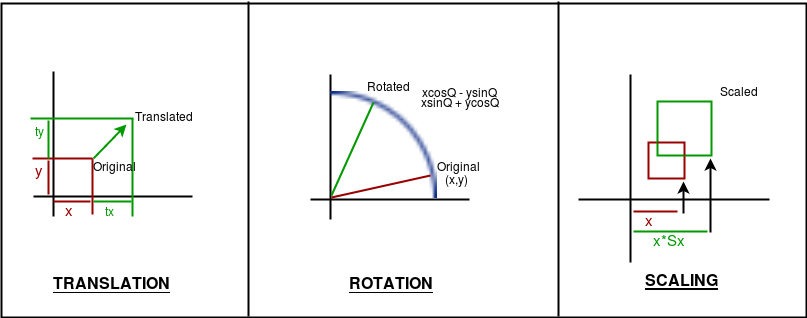Related Articles
Basic Transformations in OPENGL
• Last Updated : 08 Feb, 2018

Transformations play a very important role in manipulating objects on screen. It should be noted that here the algorithms will be implemented in code and the built-in functions will not be used to give a good understanding of how the algorithms work.
Also note that all transformations are implemented in 2D.
There are three basic kinds of Transformations in Computer Graphics:
1. Translation
2. Rotation
3. ScalingAlgorithms

1. Translation : Translation refers to moving an object to a different position on screen.
```Formula:  X = x + tx
Y = y + ty
where tx and ty are translation coordinates

The OpenGL function is glTranslatef( tx, ty, tz );```
2. Rotation : Rotation refers to rotating a point.
```Formula:  X = xcosA - ysinA
Y = xsinA + ycosA,
A is the angle of rotation.
The above formula will rotate the point around the origin.
To rotate around a different point, the formula:
X = cx + (x-cx)*cosA - (y-cy)*sinA,
Y = cx + (x-cx)*sinA + (y-cy)*cosA,
cx, cy is centre coordinates,
A is the angle of rotation.

The OpenGL function is glRotatef (A, x, y, z). ```
3. Scaling : Scaling refers to zooming in and out an object in different scales across axes.
```Formula: X = x*sx
Y = y*sy,    sx, sy being scaling factors.

The OpenGL function is glScalef(float x, float y, float z)
```

Note: If combined transformations is to be applied, follow the order: translate, rotate, scale

Implementation

 `// C code to implement basic``// transformations in OPENGL``#include ``#include ``#include ``#include `` ` `// window size``#define maxWD 640``#define maxHT 480`` ` `// rotation speed``#define thetaSpeed 0.05`` ` `// this creates delay between two actions``void` `delay(unsigned ``int` `mseconds)``{``    ``clock_t` `goal = mseconds + ``clock``();``    ``while` `(goal > ``clock``())``        ``;``}`` ` `// this is a basic init for the glut window``void` `myInit(``void``)``{``    ``glClearColor(1.0, 1.0, 1.0, 0.0);``    ``glMatrixMode(GL_PROJECTION);``    ``glLoadIdentity();``    ``gluOrtho2D(0.0, maxWD, 0.0, maxHT);``    ``glClear(GL_COLOR_BUFFER_BIT);``    ``glFlush();``}`` ` `// this function just draws a point``void` `drawPoint(``int` `x, ``int` `y)``{``    ``glPointSize(7.0);``    ``glColor3f(0.0f, 0.0f, 1.0f);``    ``glBegin(GL_POINTS);``    ``glVertex2i(x, y);``    ``glEnd();``}`` ` `void` `rotateAroundPt(``int` `px, ``int` `py, ``int` `cx, ``int` `cy)``{``    ``float` `theta = 0.0;``    ``while` `(1) {``        ``glClear(GL_COLOR_BUFFER_BIT);``        ``int` `xf, yf;`` ` `        ``// update theta anticlockwise rotation``        ``theta = theta + thetaSpeed;`` ` `        ``// check overflow``        ``if` `(theta >= (2.0 * 3.14159))``            ``theta = theta - (2.0 * 3.14159);`` ` `        ``// actual calculations..``        ``xf = cx + (``int``)((``float``)(px - cx) * ``cos``(theta))``             ``- ((``float``)(py - cy) * ``sin``(theta));``        ``yf = cy + (``int``)((``float``)(px - cx) * ``sin``(theta))``             ``+ ((``float``)(py - cy) * ``cos``(theta));`` ` `        ``// drawing the centre point``        ``drawPoint(cx, cy);`` ` `        ``// drawing the rotating point``        ``drawPoint(xf, yf);``        ``glFlush();``        ``// creating a delay``        ``// so that the point can be noticed``        ``delay(10);``    ``}``}`` ` `// this function will translate the point``void` `translatePoint(``int` `px, ``int` `py, ``int` `tx, ``int` `ty)``{``    ``int` `fx = px, fy = py;``    ``while` `(1) {``        ``glClear(GL_COLOR_BUFFER_BIT);`` ` `        ``// update``        ``px = px + tx;``        ``py = py + ty;`` ` `        ``// check overflow to keep point in screen``        ``if` `(px > maxWD || px < 0 || py > maxHT || py < 0) {``            ``px = fx;``            ``py = fy;``        ``}`` ` `        ``drawPoint(px, py); ``// drawing the point`` ` `        ``glFlush();``        ``// creating a delay``        ``// so that the point can be noticed``        ``delay(10);``    ``}``}`` ` `// this function draws``void` `scalePoint(``int` `px, ``int` `py, ``int` `sx, ``int` `sy)``{``    ``int` `fx, fy;``    ``while` `(1) {``        ``glClear(GL_COLOR_BUFFER_BIT);`` ` `        ``// update``        ``fx = px * sx;``        ``fy = py * sy;`` ` `        ``drawPoint(fx, fy); ``// drawing the point`` ` `        ``glFlush();``        ``// creating a delay``        ``// so that the point can be noticed``        ``delay(500);`` ` `        ``glClear(GL_COLOR_BUFFER_BIT);`` ` `        ``// update``        ``fx = px;``        ``fy = py;`` ` `        ``// drawing the point``        ``drawPoint(fx, fy);``        ``glFlush();``        ``// creating a delay``        ``// so that the point can be noticed``        ``delay(500);``    ``}``}`` ` `// Actual display function``void` `myDisplay(``void``)``{``    ``int` `opt;``    ``printf``(``"\nEnter\n\t<1> for translation"``           ``"\n\t<2> for rotation"``           ``"\n\t<3> for scaling\n\t:"``);``    ``scanf``(``"%d"``, &opt);``    ``printf``(``"\nGo to the window..."``);``    ``switch` `(opt) {``    ``case` `1:``        ``translatePoint(100, 200, 1, 5);``        ``break``;``    ``case` `2:``        ``rotateAroundPt(200, 200, maxWD / 2, maxHT / 2);``        ``// point will circle around``        ``// the centre of the window``        ``break``;``    ``case` `3:``        ``scalePoint(10, 20, 2, 3);``        ``break``;``    ``}``}`` ` `void` `main(``int` `argc, ``char``** argv)``{``    ``glutInit(&argc, argv);``    ``glutInitDisplayMode(GLUT_SINGLE | GLUT_RGB);``    ``glutInitWindowSize(maxWD, maxHT);``    ``glutInitWindowPosition(100, 150);``    ``glutCreateWindow(``"Transforming point"``);``    ``glutDisplayFunc(myDisplay);``    ``myInit();``    ``glutMainLoop();``}`

This article is contributed by Suprotik Dey. If you like GeeksforGeeks and would like to contribute, you can also write an article using contribute.geeksforgeeks.org or mail your article to contribute@geeksforgeeks.org. See your article appearing on the GeeksforGeeks main page and help other Geeks.

Please write comments if you find anything incorrect, or you want to share more information about the topic discussed above.My Personal Notes arrow_drop_up